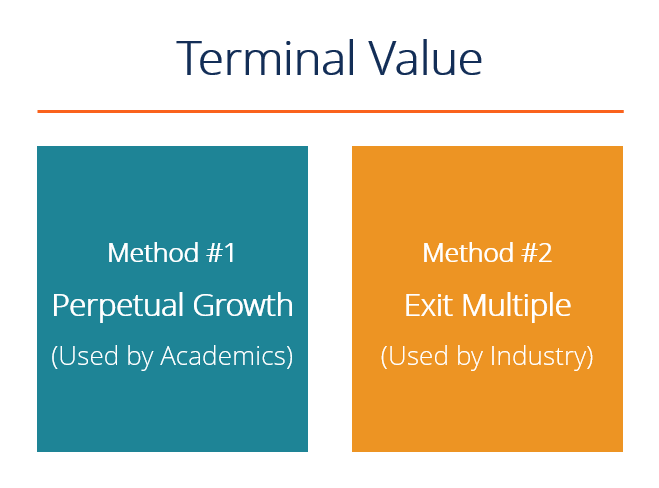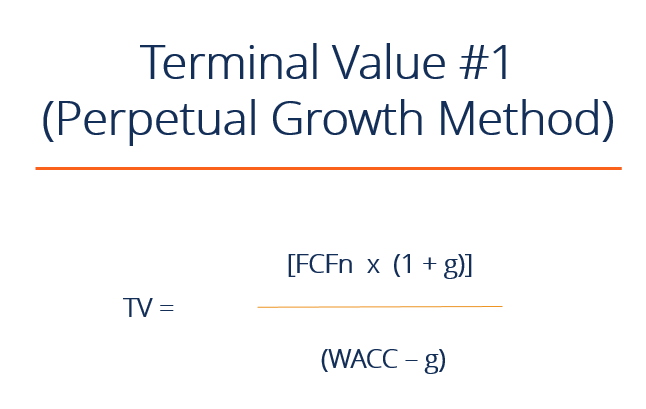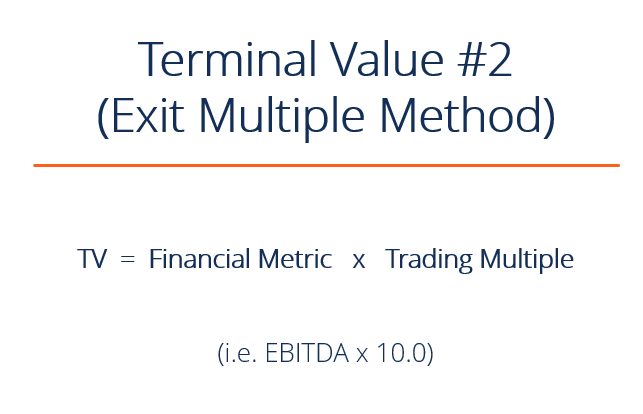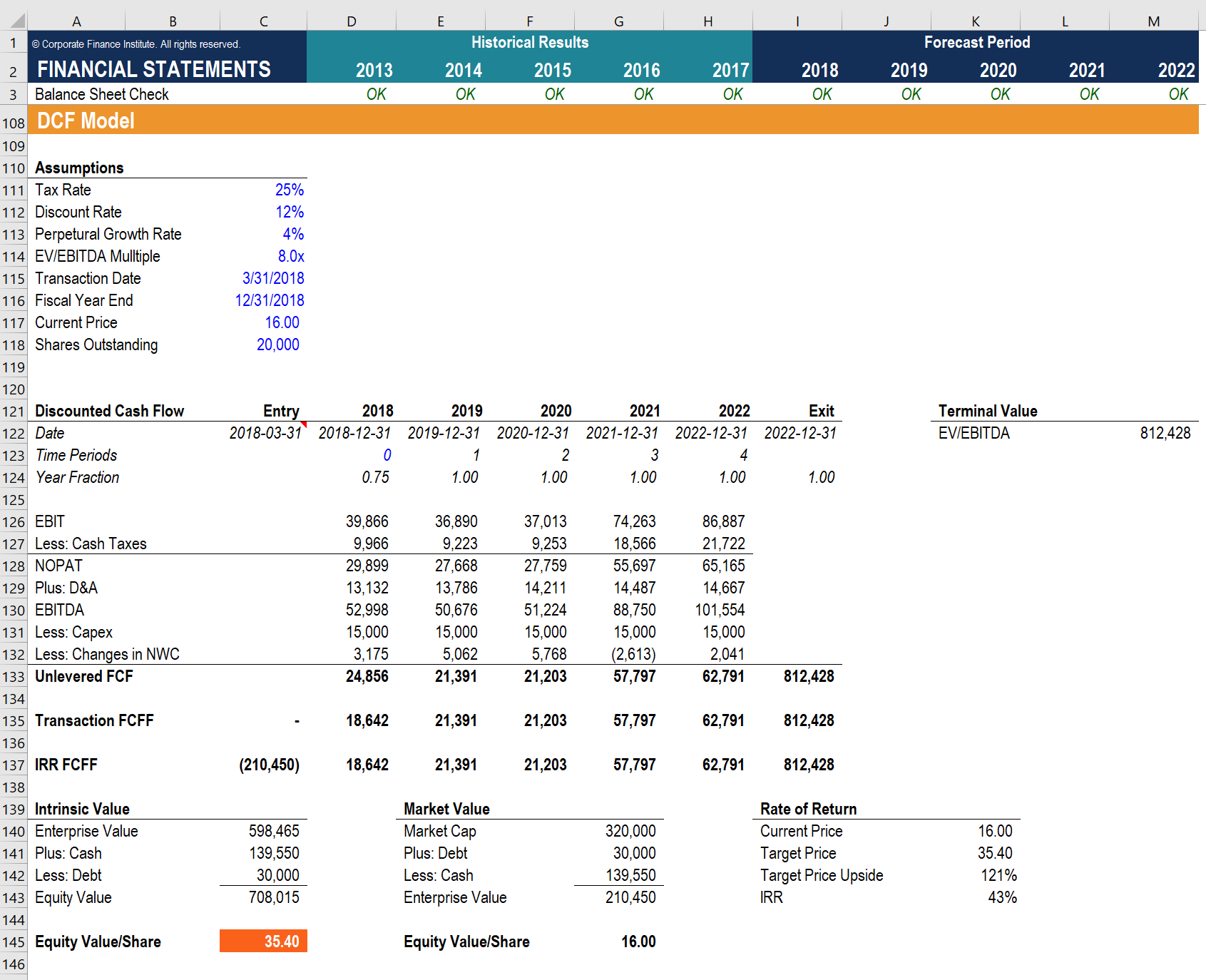# DCF Terminal Value Formula

What terminal value is, how to calculate it, and how it's used in a DCF analysis.

## What is the DCF Terminal Value Formula?

Terminal value is the estimated value of a business beyond the explicit forecast period. It is a critical part of the financial model as it typically makes up a large percentage of the total value of a business.  There are two approaches to the terminal value formula: (1) perpetual growth, and (2) exit multiple.### Why is a Terminal Value Used?

When building a Discounted Cash Flow / DCF model there are two major components: (1) the forecast period and (2) the terminal value.

The forecast period is typically 3-5 years for a normal business (but can be much longer in some types of businesses, like oil and gas or mining) because this is a reasonable amount of time to make detailed assumptions.  Anything beyond that becomes a real guessing game, which is where the terminal value comes in.

### What is the Perpetual Growth DCF Terminal Value Formula?

The perpetual growth method of calculating a terminal value formula is the preferred method among academics as it has the mathematical theory behind it.  This method assumes the business will continue to generate Free Cash Flow (FCF) at a normalized state forever (perpetuity).

The formula for calculating the terminal value is:

TV  =  (FCFn x (1 + g))  /  (WACC – g)

Where:
TV = terminal value
FCF = free cash flow
g = perpetual growth rate of FCF
WACC = weighted average cost of capital### What is the Exit Multiple DCF Terminal Value Formula?

The exit multiple approach assumes the business is sold for a multiple of some metric (i.e. EBITDA) based on currently observed comparable trading multiples for similar businesses.

The formula for calculating the terminal value is:

TV  =  Financial metric (i.e. EBITDA)  x  trading multiple (i.e. 10x)### Which Terminal Value Method is More Common?

The exit multiple approach is more common among industry professionals as they prefer to compare the value of a business to something they can observe in the market.  Among academics, you will hear more talk about the perpetual growth model since it has more theory behind it.  Some industry practitioners will take a hybrid approach and use an average of both.

### Example from a Financial Model

Below is an example of a DCF Model with a terminal value formula that uses the Exit Multiple approach.  The model assumes an 8.0x EV/EBITDA sale of the business that closes on 12/31/2022.

As you will notice, the terminal value represents a very large proportion of the total Free Cash Flow to the Firm (FCFF). In fact, it represents approximately three times as much cash flow as the forecast period. For this reason, DCF models are very sensitive to assumptions that are made about terminal value.

A common way to help represent this is through sensitivity analysis.Image: CFI’s Financial Modeling Course.

### Video Explanation of Terminal Value

Below is a short video tutorial that explains how to calculate TV step by step in Excel. This example is taken from CFI’s financial modeling courses.

### More Valuation Resources

We hope this article has helped you break down the terminal value formula between the exit multiple method and the perpetual growth method.  CFI is the official global provider of the Financial Modeling & Valuation Analyst (FMVA)® designation. To learn more about valuation and financial modeling, check out these additional resources:

• Overview of valuation methods
• DCF modeling guide